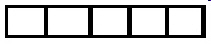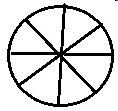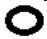3.G.2..-Test1C Partition shapes into parts with equal areas.
 Name:    3.G.2..-Test1C Partition shapes into parts with equal areas.

 CompletionComplete each statement. 1. If a shape was divided into half, what fraction would each part be? 2. In the shape below, what fraction would each part be?3. If a shape was divided into 72 equal parts, what fraction would each part be? 4. If a shape was divided into 97 equal parts, what fraction would each part be? 5. if a circle is divided like the one below, what fraction would each part be?6. Write the fraction that describes the image below.7. What is the fraction of the whole object for each section in the diagram below?8. If a shape was divided into fourths, what fraction would each part be? 9. If a shape was divided into thirds, what fraction would each part be? 10. If a shape was divided into halves, what fraction would each part be?